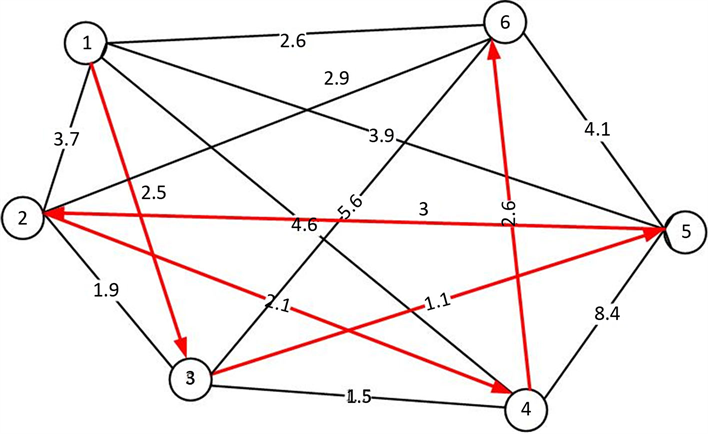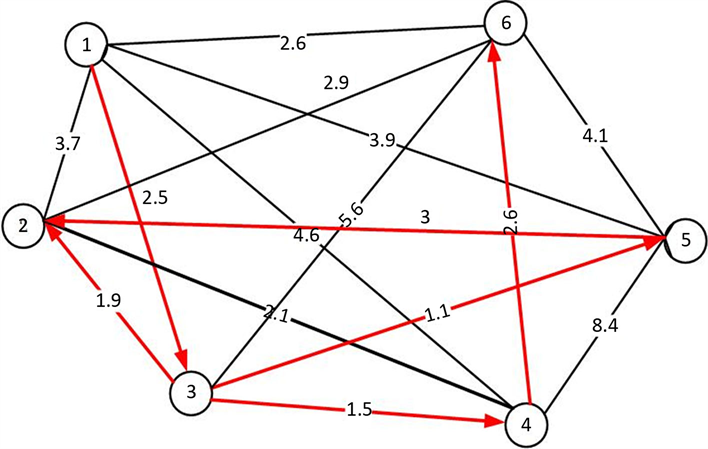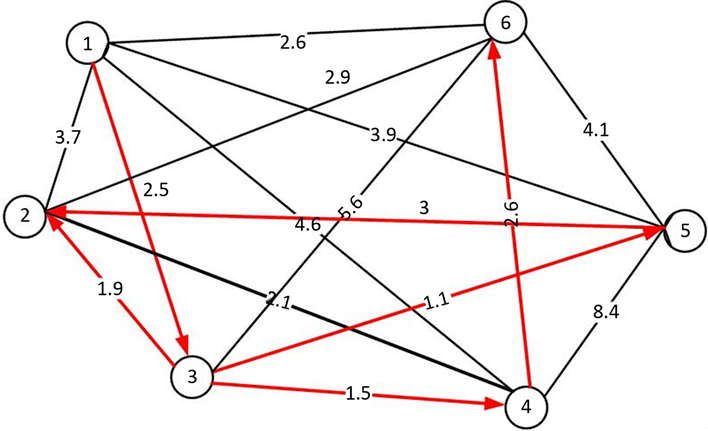﻿ 管道路线的优化设计

# 管道路线的优化设计Pipeline Route Optimization Design

Abstract: With the rapid development of industry and urbanization, the demand for tap water is increasing in urban construction, and pipeline is an important way to transport tap water. The thought of this article, based on the Dijkstra algorithm, in view of the network system of pipeline route, the shortest route optimization design, algorithm steps are given; and by using the MATLAB calculation program of optimal design for the pipeline, the shortest path is obtained. Finally, through the numerical test, the rationality and practicability of the model are further verified. The establish-ment of the model has great social and economic benefits and saves social resources.

1. 引言

2. 优化设计数学模型

2.1. 单条线路的管道铺设方案(附录1)Figure 1. Single line pipe laying diagram

$A=\left(\begin{array}{cccc}{a}_{11}& {a}_{12}& \cdot \cdot \cdot & {a}_{1n}\\ {b}_{11}& {b}_{12}& \cdot \cdot \cdot & {b}_{1n}\\ ⋮& ⋮& \ddots & ⋮\\ {b}_{n1}& {b}_{n2}& \cdot \cdot \cdot & {b}_{nn}\end{array}\right)$

$B=\left(\begin{array}{ccccccc}{a}_{11}& \cdot \cdot \cdot & {a}_{1\left(m-1\right)}& \infty & {a}_{1\left(m+1\right)}& \cdot \cdot \cdot & {a}_{1n}\\ ⋮& \ddots & ⋮& \ddots & ⋮& \ddots & ⋮\\ {b}_{\left(k+1\right)1}& \cdot \cdot \cdot & {b}_{\left(k+1\right)\left(m-1\right)}& \infty & {b}_{\left(k+1\right)\left(m+1\right)}& \cdot \cdot \cdot & {b}_{\left(k+1\right)n}\\ ⋮& \ddots & ⋮& \ddots & ⋮& \ddots & ⋮\\ {b}_{n1}& \cdot \cdot \cdot & {b}_{n\left(m-1\right)}& \infty & {b}_{n\left(m+1\right)}& \cdot \cdot \cdot & {b}_{nn}\end{array}\right)$

$C=\left(\begin{array}{ccccccc}{a}_{11}& \cdot \cdot \cdot & {a}_{1\left(p-1\right)}& \infty & {a}_{1\left(p+1\right)}& \cdot \cdot \cdot & {a}_{1n}\\ ⋮& \ddots & ⋮& \ddots & ⋮& \ddots & ⋮\\ {b}_{\left(m+1\right)1}& \cdot \cdot \cdot & {b}_{\left(m+1\right)\left(p-1\right)}& \infty & {b}_{\left(m+1\right)\left(p+1\right)}& \cdot \cdot \cdot & {b}_{\left(m+1\right)n}\\ ⋮& \ddots & ⋮& \ddots & ⋮& \ddots & ⋮\\ {b}_{n1}& \cdot \cdot \cdot & {b}_{n\left(p-1\right)}& \infty & {b}_{n\left(p+1\right)}& \cdot \cdot \cdot & {b}_{nn}\end{array}\right)$

2.2. 树状的管道铺设方案(附录2)

$A=\left(\begin{array}{cccc}{a}_{00}& {a}_{01}& \cdot \cdot \cdot & {a}_{0n}\\ {a}_{10}& {a}_{11}& \cdot \cdot \cdot & {a}_{1n}\\ ⋮& ⋮& \ddots & ⋮\\ {a}_{n0}& {a}_{n1}& \cdot \cdot \cdot & {a}_{nn}\end{array}\right)$

$B=\left(\begin{array}{ccccccc}{a}_{00}& \cdot \cdot \cdot & {a}_{0\left(k-1\right)}& \infty & {a}_{0\left(k+1\right)}& \cdot \cdot \cdot & {a}_{0n}\\ ⋮& \ddots & ⋮& ⋮& ⋮& \ddots & ⋮\\ {a}_{\left(k-1\right)0}& \cdot \cdot \cdot & {a}_{\left(k-1\right)\left(k-1\right)}& {a}_{\left(k-1\right)k}& {a}_{\left(k-1\right)\left(k+1\right)}& \cdot \cdot \cdot & {a}_{\left(k-1\right)n}\\ \infty & \cdot \cdot \cdot & {a}_{k\left(k-1\right)}& {a}_{kk}& {a}_{k\left(k+1\right)}& \cdot \cdot \cdot & {a}_{kn}\\ {a}_{\left(k+1\right)0}& \cdot \cdot \cdot & {a}_{\left(k+1\right)\left(k-1\right)}& {a}_{\left(k+1\right)k}& {a}_{\left(k+1\right)\left(k+1\right)}& \cdot \cdot \cdot & {a}_{\left(k+1\right)n}\\ ⋮& \ddots & ⋮& ⋮& ⋮& \ddots & ⋮\\ {a}_{n0}& \cdot \cdot \cdot & {a}_{n\left(k-1\right)}& {a}_{nk}& {a}_{n\left(k+1\right)}& \cdot \cdot \cdot & {a}_{nn}\end{array}\right)$Figure 2. Tree piping layout

① 若 ${a}_{0m}$ 是其中的最小值，那么表示从自来水厂直接到城市m距离最小；

② 若 ${a}_{kp}$ 是其中的最小值，那么表示从第k个城市到第p个城市之间距离最小，我们就可以从城市k直接铺设管道到达城市p，这样就会减少管道铺设的总线路，达到最节约管材。

① 若 ${a}_{0q}$ 是最小的元素，即从自来水厂直接铺设管道到城市q会使得总路线最短；

② 若 ${a}_{kw}$ 是最小的元素，即从城市k铺设管道到城市w会使得总路线最短；

③ 若 ${a}_{pr}$ 是最小的元素，即从城市p铺设管道到城市r会使得总路线最短。

3. 数值算例

Step1：用矩阵形式表示自来水厂和各个城市之间的距离以及5个城市之间的距离：Table 1. Distance between points

$A=\left(\begin{array}{cccccc}\infty & 3.7& 2.5& 4.6& 3.9& 2.6\\ 3.7& \infty & 1.9& 2.1& 3& 2.9\\ 2.5& 1.9& \infty & 1.5& 1.1& 5.6\\ 4.6& 2.1& 1.5& \infty & 8.4& 2.6\\ 3.9& 3& 1.1& 8.4& \infty & 4.1\\ 2.6& 2.9& 5.6& 2.6& 4.1& \infty \end{array}\right)$

Step2：先比较第一行元素的大小值，经过比较得出第一行中最小值为 ${a}_{13}=2.5$ ，再将矩阵A中的 ${a}_{13}$${a}_{31}$ 都用无穷大值替换，得到矩阵B：

$B=\left(\begin{array}{cccccc}\infty & 3.7& \infty & 4.6& 3.9& 2.6\\ 3.7& \infty & 1.9& 2.1& 3& 2.9\\ \infty & 1.9& \infty & 1.5& 1.1& 5.6\\ 4.6& 2.1& 1.5& \infty & 8.4& 2.6\\ 3.9& 3& 1.1& 8.4& \infty & 4.1\\ 2.6& 2.9& 5.6& 2.6& 4.1& \infty \end{array}\right)$

Step3：比较矩阵中的第一行和第三行的元素，得最小值 ${a}_{35}=1.1$ ，再将矩阵B的 ${a}_{35}$${a}_{53}$ 用无穷大值替换，得到矩阵C：

$C=\left(\begin{array}{cccccc}\infty & 3.7& \infty & 4.6& 3.9& 2.6\\ 3.7& \infty & 1.9& 2.1& 3& 2.9\\ \infty & 1.9& \infty & 1.5& \infty & 5.6\\ 4.6& 2.1& 1.5& \infty & 8.4& 2.6\\ 3.9& 3& \infty & 8.4& \infty & 4.1\\ 2.6& 2.9& 5.6& 2.6& 4.1& \infty \end{array}\right)$Figure 3. Pipe laying diagram

Step4：比较矩阵中的第一行、第三行和第五行元素，得最小值是 ${a}_{34}=1.5$ ，再将矩阵C中的 ${a}_{34}$${a}_{43}$ 用无穷大值替换，得到矩阵D：

$D=\left(\begin{array}{cccccc}\infty & 3.7& \infty & 4.6& 3.9& 2.6\\ 3.7& \infty & 1.9& 2.1& 3& 2.9\\ \infty & 1.9& \infty & \infty & \infty & 5.6\\ 4.6& 2.1& \infty & \infty & 8.4& 2.6\\ 3.9& 3& \infty & 8.4& \infty & 4.1\\ 2.6& 2.9& 5.6& 2.6& 4.1& \infty \end{array}\right)$

Step5：依次进行下去，就可以得到管道铺设方案为从自来水厂铺设管道到第3个城市，再从第3个城市铺设管道到达第5个城市，再由第3个城市铺设管道到第4个城市，由第3个城市铺设管道到第2个城市，再由第4个城市铺设管道到第6个城市，总长为 $9.6×{10}^{5}\text{m}$ ，分布图如图3所示。

function [U,V,d]=lcxz(A,b)

B=A;

U=zeros(1,n);

V=ones(1,n);

R=b*ones(1,n);

L=b*ones(m,1);

a=A(1,:);

B(1,:)=R;

B(:,1)=L;

t=1;

U(1,2)=u;

V(1,2)=v;

for i=2:n-1

s=v;

f=B(v,:);

t=i+t;

U(1,i+1)=u;

V(1,i+1)=v;

B(s,:)=R;

B(:,s)=L;

i=i+1;

end

U;

V;

d=sum(U);

function [U,V,d]=ndjtsl(A,b)

C=inf*ones(n);

B=A;

U=zeros(1,n);

V=ones(1,n);

rep=b;

a=B(1,:);

B(1,v)=rep;

B(v,1)=rep;

U(1,2)=u;

V(1,2)=v;

f=B(1,:);

for i=3:n

r=v;

F=[f;B(v,:)];

rv=v;

U(1,i)=u;

x=v;

s=V(x);

y=rv(v);

v=y;

V(1,i)=y;

B(r,y)=rep;

B(y,r)=rep;

B(s,y)=rep;

B(y,s)=rep;

f=[f;B(r,:)];

f(x,y)=rep;

i=i+1;

end

d=sum(U);

 王俊珺, 夏华丽, 等. 物流配送路线规划中的最短路径研究[J]. 农业网络信息, 2007(5): 60-62.

 施益昌, 郑贤斌, 等. 基于MATLAB动态规划中最短路线的实现程序[J]. 电脑学习, 2003(6): 37-38.

 赵娟, 王建新. 用动态规划方法求解最短运输路线问题[J]. 现代电子技术, 2012, 35(17): 120-122.

 张水舰, 刘学军, 杨洋. 动态随机最短路径算法研究[J]. 物理学报, 2012, 61(16): 7-16.

 王树西, 吴政学. 改进的Dijkstra最短路径算法及其应用研究[J]. 计算机科学, 2012, 5(39): 223-228.

 王荣, 江东, 韩惠. 基于Floyd方法的最短路径算法优化算法[J]. 甘肃科学报, 2012, 4(24): 114-118.

Top